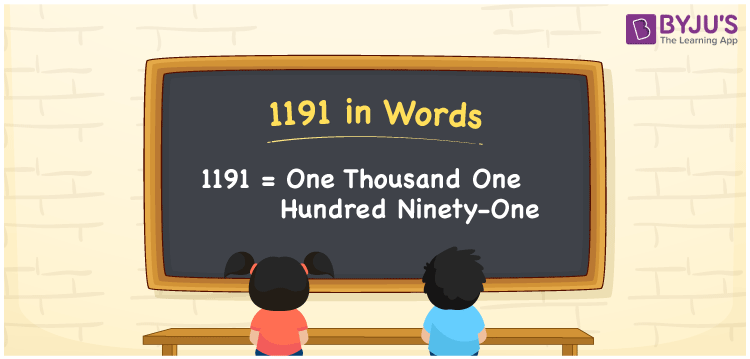# 1191 in Words

One thousand one hundred ninety-one is the number name for the numeral 1191. If you purchased the notebooks for Rs. 1191, it can be written as “I purchased the notebooks for Rs. One thousand one hundred ninety-one. The number 1191 is a 4 digit number and it appears after 1190 but before 1192. This article will guide you to learn the process of writing the number 1191 in words using the place value system.

 1191 in Words: One Thousand One Hundred Ninety-one. One Thousand One Hundred Ninety-one in Numerical Form: 1191.

## 1191 in English Words## How to Write 1191 in Words?

Learn the place values of 1191 to write the number in words.

 Thousands Hundreds Tens Ones 1 1 9 1

The expanded form of 1191 is as follows:

= 1 × Thousand + 1 × Hundred + 9 × Ten + 1 × One

= 1 × 1000 + 1 × 100 + 9 × 10 + 1 × 1

= 1000 + 100 + 90 + 1

= 1191

= One thousand one hundred ninety-one

Hence, 1191 in words is one thousand one hundred ninety-one.

1191 in words – One thousand one hundred ninety-one

Is 1191 an odd number? – Yes

Is 1191 an even number? – No

Is 1191 a perfect square number? – No

Is 1191 a perfect cube number? – No

Is 1191 a prime number? – No

Is 1191 a composite number? – Yes

## Frequently Asked Questions on 1191 in Words

### Write 1191 in words.

1191 in words is one thousand one hundred ninety-one.

### Simplify 1100 + 91, and express it in words.

Simplifying 1100 + 91, we get 1191. Hence, 1191 in words is one thousand one hundred ninety-one.

### Is 1191 an odd number?

Yes, 1191 is an odd number.